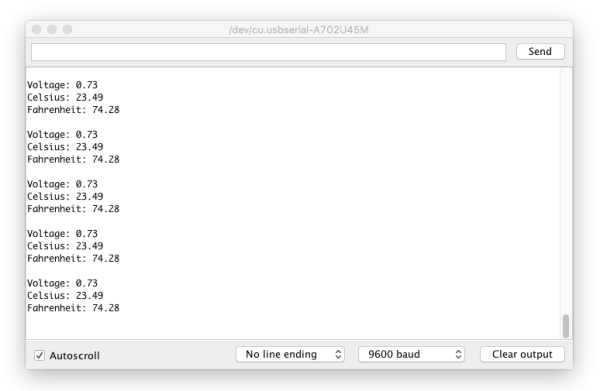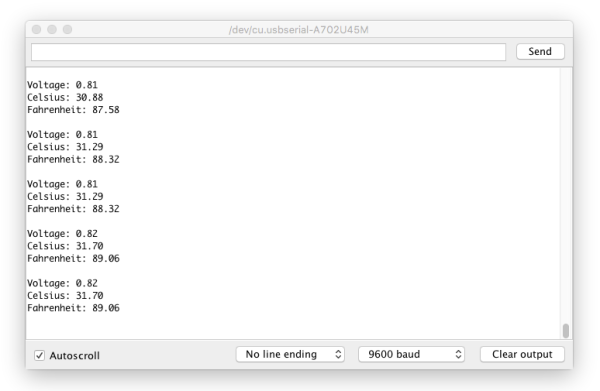# LilyPad Development Board Activity Guide

Pages

This activity will use another kind of analog sensor, the temperature sensor. We'll create a thermal alert program which lights up the channels of the tri-color LED if it senses 'hot' or 'cold'. We'll also add in the LilyPad Vibe Board to provide some haptic feedback.

### LilyPad Boards Used in This Activity

What is a Temperature Sensor?

The LilyPad Temperature Sensor outputs voltage depending on the ambient temperature around it. Similar to the light sensor, the temperature sensor has three sew tabs - positive, negative, and signal (S). The temperature sensor board will output specific voltage at set temperatures - 10mV for every degree Celsius (C), with 0 degrees C being set at 0.5V. The current flowing from the board through the signal tab to your LilyPad Arduino Simple can be converted through a formula to degrees in Celsius or Fahrenheit.

What is a Vibration Motor?

The LilyPad Vibe Board is a small motor that shakes when current is applied to it. This board can be used in wearable applications to provide simple haptic feedback.

### New Concepts Introduced in This Activity

#### Multiple Thresholds:

In this activity, we'll use thresholds like the Night-Light project, but assign multiple values associated with hot and cold. We'll use comparison operators to determine if the temperature is within the bounds of each threshold.

#### Temperature Conversion

The temperature sensor produces an analog voltage representing the temperature near it. In order to get readings in degrees, we'll have to do some math. The voltage output from the sensor is linearly proportional to the Celsius temperature. Once you know the output voltage of the sensor, you can calculate the temperature with this equation:

To convert that reading to Fahrenheit, use this formula:

Next we'll be use Serial Monitor to read the values coming from the sensor and insert the formulas in our code to display the temperature in both Celsius and Fahrenheit.

### Example Code

To open the code, go to:

You can also copy and paste the following code into the Arduino IDE. Hit upload, and see what happens!

``````language:c
/*
SparkFun Electronics
https://www.sparkfun.com/products/11262

This code reads the input of the temperature sensor and compares it to
a two set thresholds named 'hotAlert' and 'coldAlert'. If temperature is above
the hotAlert threshold, the red channel of the tri-color LED will turn on and the vibe board
will shake. If the temperature falls below the coldAlert threshold, the blue channel of the
tri-color LED will turn on. If the temperature is in the middle of these two thresholds,
the green channel of the tri-color LED will turn on.

This example is based on Thermal Alert! example in the Digital Sandbox:

******************************************************************************/

// Set hot and cold threshold variables to check against. If the temperature reading is above
// this number in degrees Fahrenheit, we'll turn different LEDs on
float hotAlert = 80.0;  // 80 degrees Fahrenheit
float coldAlert = 70.0; // 70 degrees Fahrenheit

// Temperature sensor is connected to A1
int sensorPin = A1;

// The LEDs in the tri-color LED
int RGB_red = 9;
int RGB_green = 11;
int RGB_blue = 10;

// The vibe board
int motor = 3;

void setup()
{
// Set the temperature sensor pin as an INPUT:
pinMode(sensorPin, INPUT);

// Make all of our LED pins outputs:

pinMode(RGB_red, OUTPUT);
pinMode(RGB_green, OUTPUT);
pinMode(RGB_blue, OUTPUT);

pinMode(motor, OUTPUT);

// Initialize Serial, set the baud rate to 9600 bps.
Serial.begin(9600);
}

void loop()
{
// Variable to store raw temperature
long rawTemp;

// Variable to store voltage calculation
float voltage;

// Variable to store Fahrenheit value
float fahrenheit;

// Variable to store Celsius value
float celsius;

// Read the raw 0-1023 value of temperature into a variable.

// Calculate the voltage, based on that value.
// Multiply by maximum voltage (4.2V for USB power) and divide by maximum ADC value (1023).
voltage = rawTemp * (4.2 / 1023.0);
Serial.print("Voltage: "); // Print voltage reading to serial monitor
Serial.println(voltage);

// Calculate the celsius temperature, based on that voltage..
celsius = (voltage - 0.5) * 100;
Serial.print("Celsius: "); // Print celcius temp to serial monitor
Serial.println(celsius);

// Use a common equation to convert celsius to Fahrenheit. F = C*9/5 + 32.
fahrenheit = (celsius * 9.0 / 5.0) + 32.0;
Serial.print("Fahrenheit: "); // Print Fahrenheit temp to serial monitor
Serial.println(fahrenheit);
// Print a blank line
Serial.println();

// Check the temperature, and turn on the LEDs associated with the hot or cold thresholds
if (fahrenheit >= hotAlert) // If the temperature rises above the hot threshold:
{
digitalWrite(RGB_red, LOW);    // Turn the red LED on
digitalWrite(RGB_green, HIGH); // Turn the green LED off
digitalWrite(RGB_blue, HIGH);  // Turn the blue LED off
digitalWrite(motor, HIGH);     // Turn the motor on to shake as an extra alert
delay(500);                    // Wait 1/2 second
digitalWrite(motor, LOW);      // Then turn the motor off
}
else if (fahrenheit < coldAlert) // If the temperature falls below the cold threshold:
{
digitalWrite(RGB_red, HIGH);   // Turn the red LED off
digitalWrite(RGB_green, HIGH); // Turn the green LED off
digitalWrite(RGB_blue, LOW);   // Turn the blue LED on
}
else // For all other temperature readings (between hot and cold):
{
digitalWrite(RGB_red, HIGH);  // Turn the red LED off
digitalWrite(RGB_green, LOW); // Turn the green LED on
digitalWrite(RGB_blue, HIGH); // Turn the blue LED off
}

// Wait 1 second between readings
delay(1000);
}
``````

### What You Should See

Open the serial monitor and press your hand on the temperature sensor to see a reading. Your body temperature may not produce a substantial change in the readings from the sensor. Try holding warm or cold objects hear the sensor (be careful with any condensation from a cold object that it does not drip onto the boards). If the reading is above the 'hot' threshold, the red LED on the tri-color LED will light up and the vibe motor will briefly shake. If the reading is below the 'cold' threshold, the blue LED on the tri-color LED will light up. If the reading is between these two thresholds, the green LED on the tri-color will light up.

If you have a certain temperature range in mind, adjust the threshold variables in your code.

#### Program Overview

1. Store the temperature level in the variable `rawTemp`.
2. Print the calculated readings to the Serial Monitor.
3. Turn on the LED color based on the hot and cold thresholds. If the temperature is hot, the vibe motor will turn on for a moment to provide feedback.
4. Repeat.

#### Observe Readings in the Serial Monitor:

Open the serial monitor to observe the ambient temperature readings converted from the sensor's voltage output.Ways to change the readings: cover the sensor with your hand or breathe on it, move something hot or cold near it.The values on your screen may look different than in this image. The range of readings will depend on your particular surroundings.

If you are having trouble seeing the values, double check that 9600 baud is selected in the dropdown menu at the bottom right of the window and the auto scroll option is checked.

#### Code to Note

CodeDescription
`float hotAlert = 80.0;`
` float coldAlert = 70.0;`
##### Set Thresholds:
In this program, two thresholds are set for hot and cold readings. Adjust these as needed for the interaction you would like in your project.
`long rawTemp;`
`float voltage;`
`float fahrenheit;`
`float celsius;`
##### Variable Data Types:
Up until this point, most of the variables in the examples have been integers (`int`). In this program, we'll use a `long` to store the raw temperature readings and floating point (`float`), which is a number with a decimal point.

`rawTemp = analogRead(sensorPin);`

`voltage = rawTemp * (4.2 / 1023.0);`
`Serial.print("Voltage: ");`
`Serial.println(voltage);`
First, the program stores the sensor reading into a variable called `rawTemp` to use in the conversion formulas. Since we know from the sensor's datasheet that voltage being read from the sensor has a relationship to temperature, we can use the `voltage = rawTemp * (4.2 / 1023.0)` formula to interpret the analog to digital converter's output and determine the voltage.

`celsius = (voltage - 0.5) * 100;`
`Serial.print("Celsius: ");`
`Serial.println(celsius);`

`float fahrenheit = (celsius * 9.0 / 5.0) + 32.0;`
`Serial.print("Fahrenheit: ");`
`Serial.println(fahrenheit);`
`Serial.println();`
##### Conversion Formulas:
Next, the code uses the voltage to translate to temperature in Celsius. Then a standard formula for converting Celsius to Fahrenheit (F = C*9/5 + 32). The program also prints all the variables to the serial monitor so you can observe them as they change. This is a great way to see the room's ambient temperature using the development board and adjust the temperature thresholds for your alerts as needed.
```if (fahrenheit >= hotAlert) { } else if (fahrenheit < coldAlert) { } else { }```
A set of `if` statements check if the `fahrenheit` variable is above, below, or between the hot & cold thresholds. As a result, the LilyPad Arduino Simple turns the red, green, and blue LEDs on depending on which threshold the variable is within. In the first `if` statement, there is also code to turn on the LilyPad Vibe Board to shake if things are too hot.

Note that the second check uses an `else if` instead of just an `if` statement to check the temperature. This is because the temperature sensor cannot be hot and cold at the same time. Using three individual `if` statements would be inefficient since the Arduino would check each condition. It is not recommended in this case since the sensor will only be able to provide one temperature value at a time. Since all three condition statements cannot be true at the same time, the test is mutually exclusive and requires each test to be grouped together.

### Coding Challenges

• Can you change the code so that the buzzer sounds different audio alerts depending on the temperature?

• Can you use the row of white LEDs as a bar graph to get more specific visual indication of the temperature variations?

• Try creating a 'quiet' mode by using the switch to silence the vibe board alarm and just display on the tri-color LED.